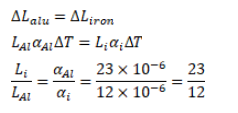# Find the ratio of lengths of an iron rod and an aluminium rodQuestion:

Find the ratio of lengths of an iron rod and an aluminium rod for which the difference in the lengths is independent of temperature. Coefficients of linear expansion of iron and aluminium are $12 \times 10^{-6} /{ }^{\circ} \mathrm{C}$ and $23 \times 10^{-6} /{ }^{\circ} \mathrm{C}$ respectively.

Solution: